# Plotting with `mne.viz.Brain`#

In this example, we’ll show how to use `mne.viz.Brain`.

```# Author: Alex Rockhill <aprockhill@mailbox.org>
#
```

In this example we use the `sample` data which is data from a subject being presented auditory and visual stimuli to display the functionality of `mne.viz.Brain` for plotting data on a brain.

```import matplotlib.pyplot as plt
from matplotlib.cm import ScalarMappable
from matplotlib.colors import Normalize

import mne
from mne.datasets import sample

print(__doc__)

data_path = sample.data_path()
subjects_dir = data_path / "subjects"
sample_dir = data_path / "MEG" / "sample"
```

Plot source information.

```brain_kwargs = dict(alpha=0.1, background="white", cortex="low_contrast")
brain = mne.viz.Brain("sample", subjects_dir=subjects_dir, **brain_kwargs)

stc.crop(0.09, 0.1)

kwargs = dict(
fmin=stc.data.min(),
fmax=stc.data.max(),
alpha=0.25,
smoothing_steps="nearest",
time=stc.times,
)
```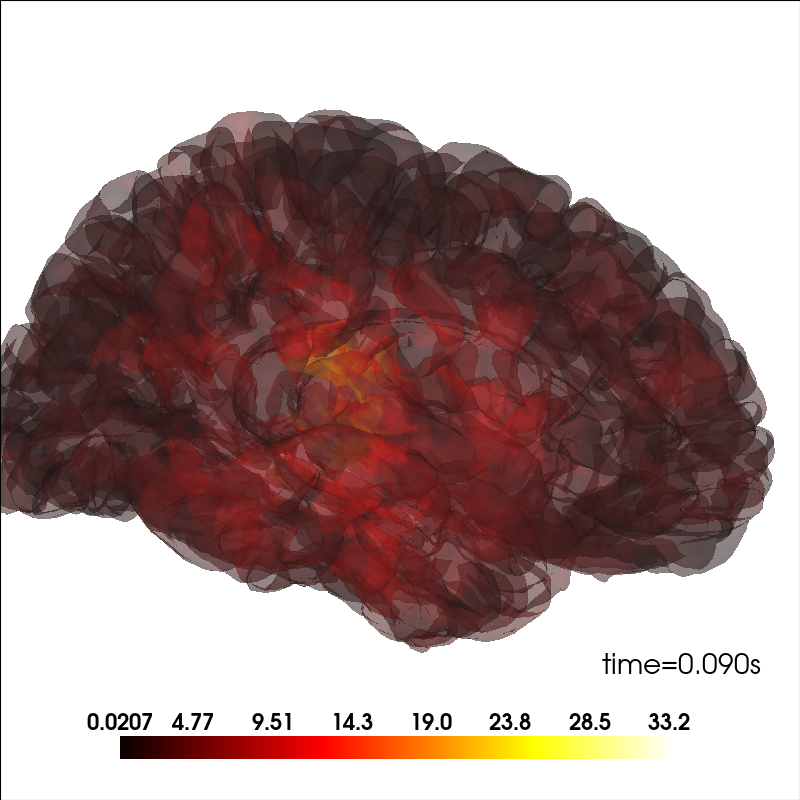## Modify the view of the brain#

You can adjust the view of the brain using `show_view` method.

```brain = mne.viz.Brain("sample", subjects_dir=subjects_dir, **brain_kwargs)
brain.show_view(azimuth=190, elevation=70, distance=350, focalpoint=(0, 0, 20))
```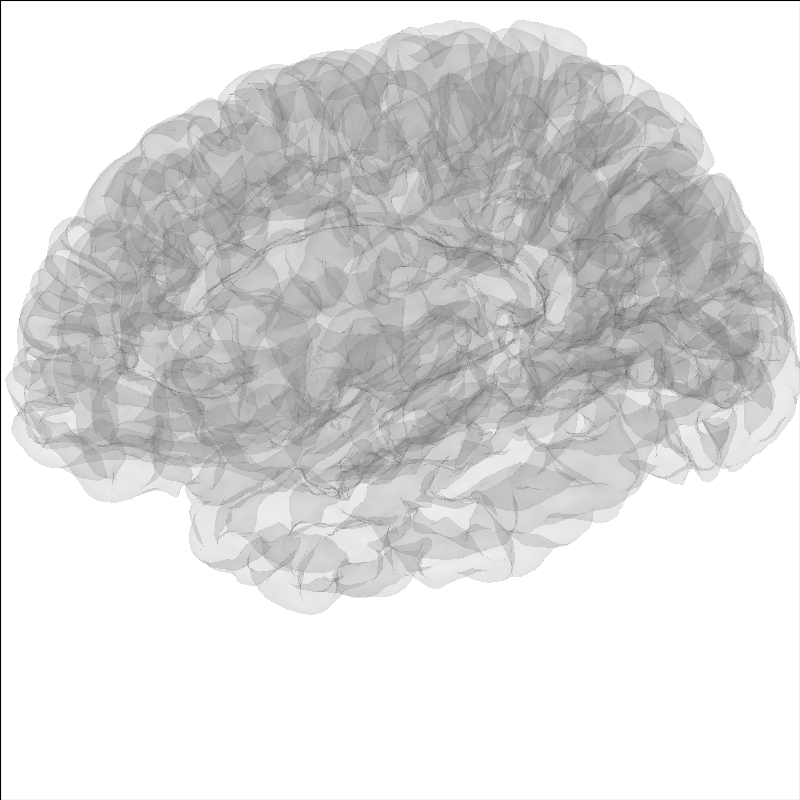## Highlight a region on the brain#

It can be useful to highlight a region of the brain for analyses. To highlight a region on the brain you can use the `add_label` method. Labels are stored in the Freesurfer label directory from the `recon-all` for that subject. Labels can also be made following the Freesurfer instructions Here we will show Brodmann Area 44.

Note

The MNE sample dataset contains only a subselection of the Freesurfer labels created during the `recon-all`.

```brain = mne.viz.Brain("sample", subjects_dir=subjects_dir, **brain_kwargs)
brain.show_view(azimuth=190, elevation=70, distance=350, focalpoint=(0, 0, 20))
```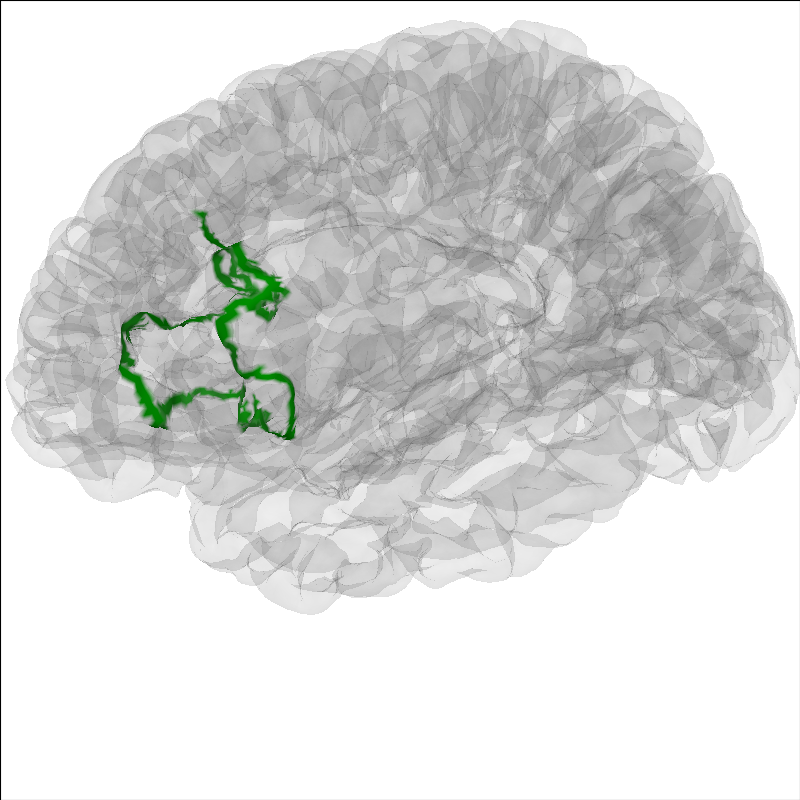## Include the head in the image#

Add a head image using the `add_head` method.

```brain = mne.viz.Brain("sample", subjects_dir=subjects_dir, **brain_kwargs)
```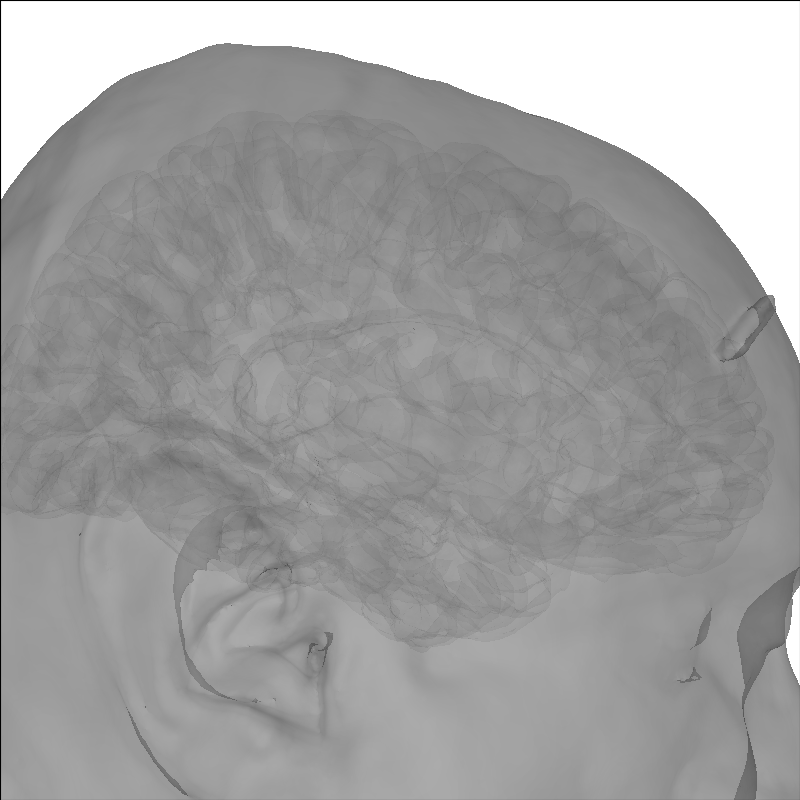```Using lh.seghead for head surface.
```

To put into context the data that generated the source time course, the sensor positions can be displayed as well.

```brain = mne.viz.Brain("sample", subjects_dir=subjects_dir, **brain_kwargs)
brain.show_view(distance=500)  # move back to show sensors
```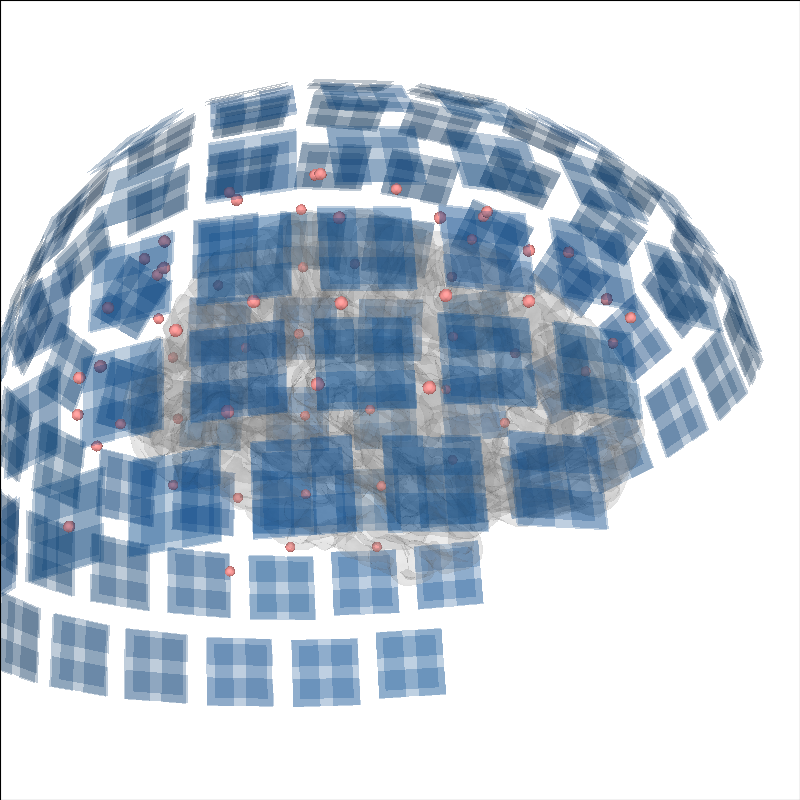```Reading /home/circleci/mne_data/MNE-sample-data/MEG/sample/sample_audvis-ave.fif ...
Read a total of 4 projection items:
PCA-v1 (1 x 102) active
PCA-v2 (1 x 102) active
PCA-v3 (1 x 102) active
Average EEG reference (1 x 60) active
Found the data of interest:
t =    -199.80 ...     499.49 ms (Left Auditory)
0 CTF compensation matrices available
nave = 55 - aspect type = 100
Projections have already been applied. Setting proj attribute to True.
No baseline correction applied
Read a total of 4 projection items:
PCA-v1 (1 x 102) active
PCA-v2 (1 x 102) active
PCA-v3 (1 x 102) active
Average EEG reference (1 x 60) active
Found the data of interest:
t =    -199.80 ...     499.49 ms (Right Auditory)
0 CTF compensation matrices available
nave = 61 - aspect type = 100
Projections have already been applied. Setting proj attribute to True.
No baseline correction applied
Read a total of 4 projection items:
PCA-v1 (1 x 102) active
PCA-v2 (1 x 102) active
PCA-v3 (1 x 102) active
Average EEG reference (1 x 60) active
Found the data of interest:
t =    -199.80 ...     499.49 ms (Left visual)
0 CTF compensation matrices available
nave = 67 - aspect type = 100
Projections have already been applied. Setting proj attribute to True.
No baseline correction applied
Read a total of 4 projection items:
PCA-v1 (1 x 102) active
PCA-v2 (1 x 102) active
PCA-v3 (1 x 102) active
Average EEG reference (1 x 60) active
Found the data of interest:
t =    -199.80 ...     499.49 ms (Right visual)
0 CTF compensation matrices available
nave = 58 - aspect type = 100
Projections have already been applied. Setting proj attribute to True.
No baseline correction applied
Channel types:: grad: 203, mag: 102, eeg: 59
Getting helmet for system 306m
```

Dipole modeling as in The role of dipole orientations in distributed source localization can be plotted on the brain as well.

```brain = mne.viz.Brain("sample", subjects_dir=subjects_dir, **brain_kwargs)
cmap = plt.colormaps["YlOrRd"]
colors = [cmap(gof / dip.gof.max()) for gof in dip.gof]
brain.add_dipole(dip, trans, colors=colors, scales=list(dip.amplitude * 1e8))
brain.show_view(azimuth=-20, elevation=60, distance=300)
img = brain.screenshot()  # for next section
```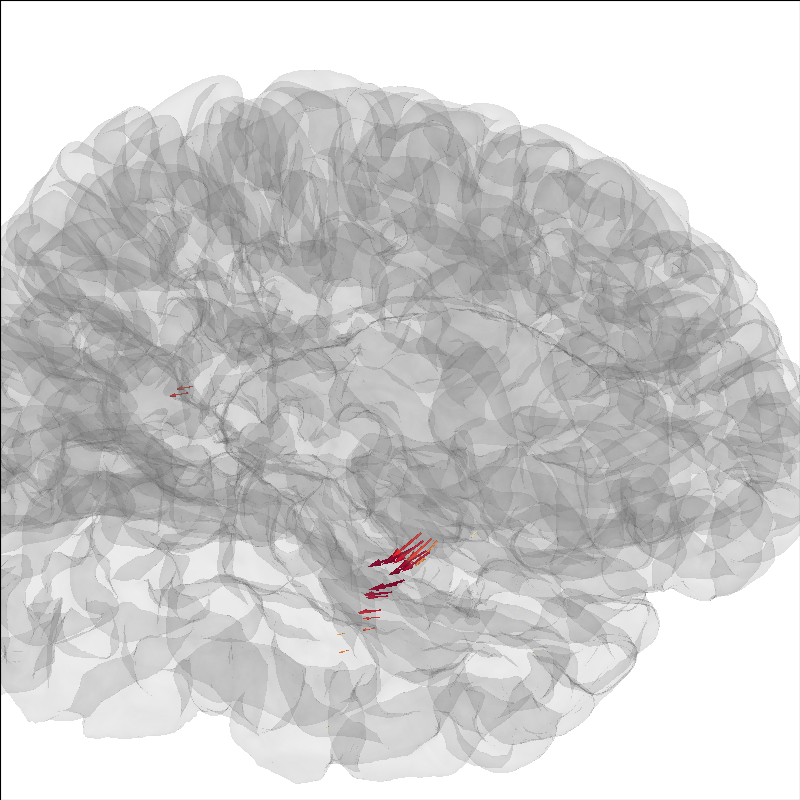```34 dipole(s) found
```

## Create a screenshot for exporting the brain image#

Also, we can a static image of the brain using `screenshot` (above), which will allow us to add a colorbar. This is useful for figures in publications.

```fig, ax = plt.subplots()
ax.imshow(img)
ax.axis("off")
cax = fig.add_axes([0.9, 0.1, 0.05, 0.8])
norm = Normalize(vmin=0, vmax=dip.gof.max())
fig.colorbar(ScalarMappable(norm=norm, cmap=cmap), cax=cax)
fig.suptitle("Dipole Fits Scaled by Amplitude and Colored by GOF")
```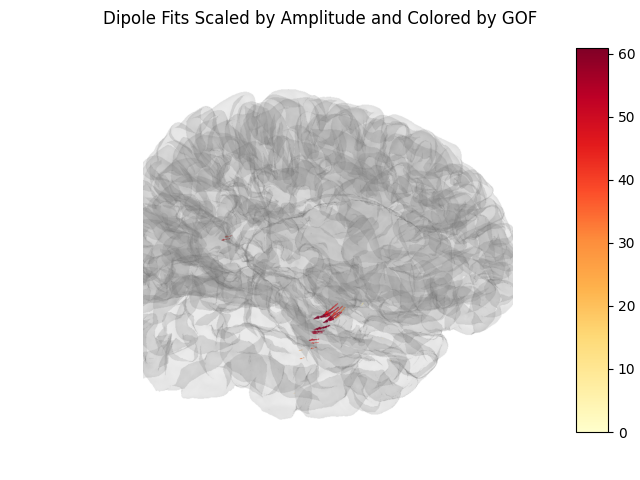Total running time of the script: (0 minutes 42.219 seconds)

Estimated memory usage: 25 MB

Gallery generated by Sphinx-Gallery